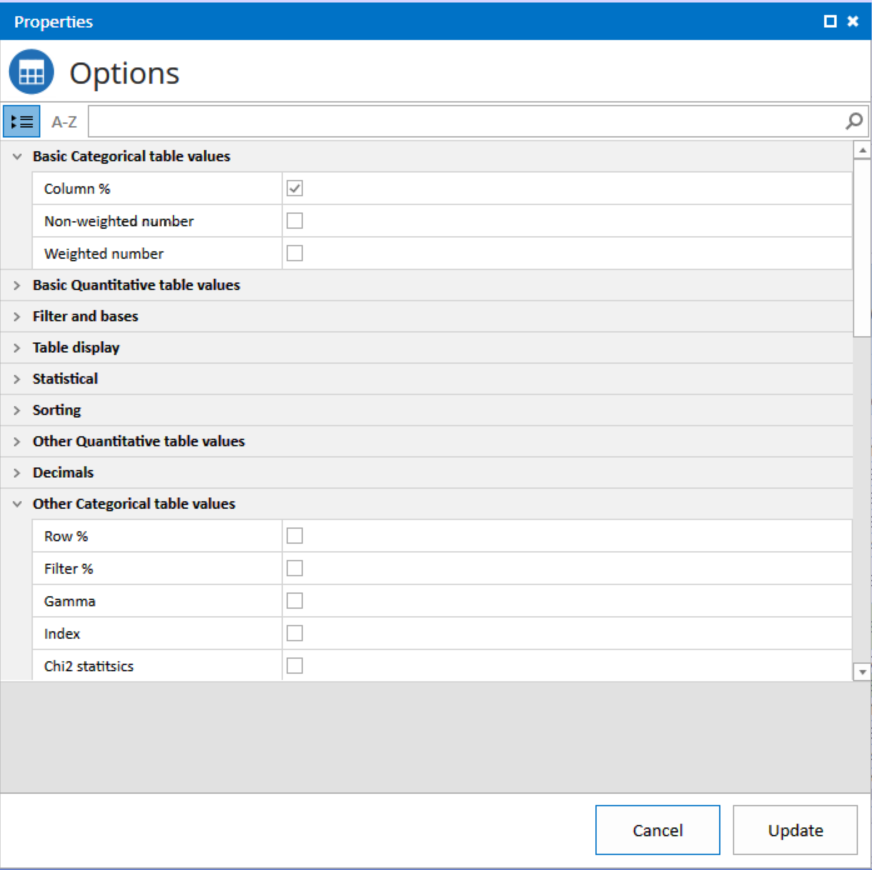# How do I change the values to be shown in cross tables?

Superdig can show different values in the cross tables depending on your specifications. System default is to show Vertical percentages only for Categorical questions and average for quantitative questions. But you may very well change this by adding, changing or removing these value types in the options menu.

To make it simple for you, we have organized the value types in 4 different categories, each explained below:

 Checkbox Label Description Basic Categorical values Row % Percentage of the row total. Column % Percentage of the column total. Non-Weighted Number Non-weighted number Weighted number Weighted population Basic Quantitative values Average Average Standard Deviation Standard deviation Other Categorical values Row % Percentage of the row total. Filter % Percentage of the total for the entire table, if there is no filter on the table. If there is a filter, then this is the percentage of the group that passes the filter for the table. Gamma Gamma value Index Categorical index.  Index is a measure of how close to the expected value the cell value is. Chi2 statistics Chi2 statistics Chi2 distribution Chi2 distribution Other Quantitative values AutoCode Automatically splits a quantitative question into discrete values or ranges of values. The system chooses the intervals based on the minimum and maximum values present. Auto code interval Default = 0, specify interval if other Row % Percentage of the row total that the cell value represents for that column group/hierarchical combination. Column % Percentage of the column total that the cell value represents for that table group. Filter % Percentage of the filter total that the cell value represents for that cell. Row SUM % Horizontal sum percentage Column SUM % Vertical sum percentage SUM Accumulated sum of all answers. Non-Weighted Number Non-weighted number Weighted number Weighted population Minimum The minimum value Maximum The maximum value Median The median value Lower Quartile The lower 25% Upper quartile The upper 25% Fractile Specify a free fractile, e.g. 33 Standard ErrorThe value of the standard Error

Note: If you want to change this permanently, i.e. you want to use these options as Your defaults, you may change these using the “global options” button on the right hand side.

Also note that in the options dialog, you can search/filter options by just typing into the earch field.